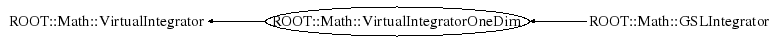# class ROOT::Math::VirtualIntegratorOneDim: public ROOT::Math::VirtualIntegrator

```
VirtualIntegrator abstract class.
Interface defining the common methods for the
numerical integrator classes of one and multi dimensions
The derived class VirtualIntegratorOneDim defines the methods
for one-dimensional integration.
The derived class VirtualIntegratorMultiDim defines the method for
multi-dimensional integration.
The concrete classes for one dimension (e.g. GSLIntegrator) or
multi-dimension (e.g. GSLMCIntegrator) can be created using the
plug-in manager

@ingroup  Integration

```

## Function Members (Methods)

This is an abstract class, constructors will not be documented.
Look at the header to check for available constructors.

public:
 virtual ~VirtualIntegratorOneDim() virtual double ROOT::Math::VirtualIntegrator::Error() const virtual double Integral() virtual double Integral(const vector& pts) virtual double Integral(double a, double b) virtual double IntegralCauchy(double a, double b, double c) virtual double IntegralLow(double b) virtual double IntegralUp(double a) ROOT::Math::VirtualIntegratorOneDim& operator=(const ROOT::Math::VirtualIntegratorOneDim&) virtual double ROOT::Math::VirtualIntegrator::Result() const virtual void ROOT::Math::VirtualIntegrator::SetAbsTolerance(double) virtual void SetFunction(const ROOT::Math::IGenFunction&, bool copy = false) virtual void ROOT::Math::VirtualIntegrator::SetRelTolerance(double) virtual int ROOT::Math::VirtualIntegrator::Status() const

## Class Charts## Function documentation

virtual ~VirtualIntegratorOneDim()
``` destructor: no operation
```
`{}`
double Integral(double a, double b)
``` evaluate integral
```
void SetFunction(const ROOT::Math::IGenFunction& , bool copy = false)
``` set integration function (flag control if function must be copied inside)
```
double Integral(const vector<double>& pts)
``` evaluate un-defined  integral (between -inf, + inf)
```
double IntegralUp(double a)
``` evaluate integral over the (a, +inf)
```
double IntegralLow(double b)
``` evaluate integral over the (-inf, b)
```
double Integral(const vector<double>& pts)
``` evaluate integral with singular points
```
double IntegralCauchy(double a, double b, double c)
``` evaluate Cauchy integral
```

Author: Magdalena Slawinska 10/2007
Last update: root/mathcore:\$Id\$
Copyright (c) 2007 LCG ROOT Math Team, CERN/PH-SFT *

This page has been automatically generated. If you have any comments or suggestions about the page layout send a mail to ROOT support, or contact the developers with any questions or problems regarding ROOT.